Calculus II

Day 18-Lecture 35

6 April 2017, Thursday 8:40

Previous Screenshot       Next Screenshot

Spherical Coordinates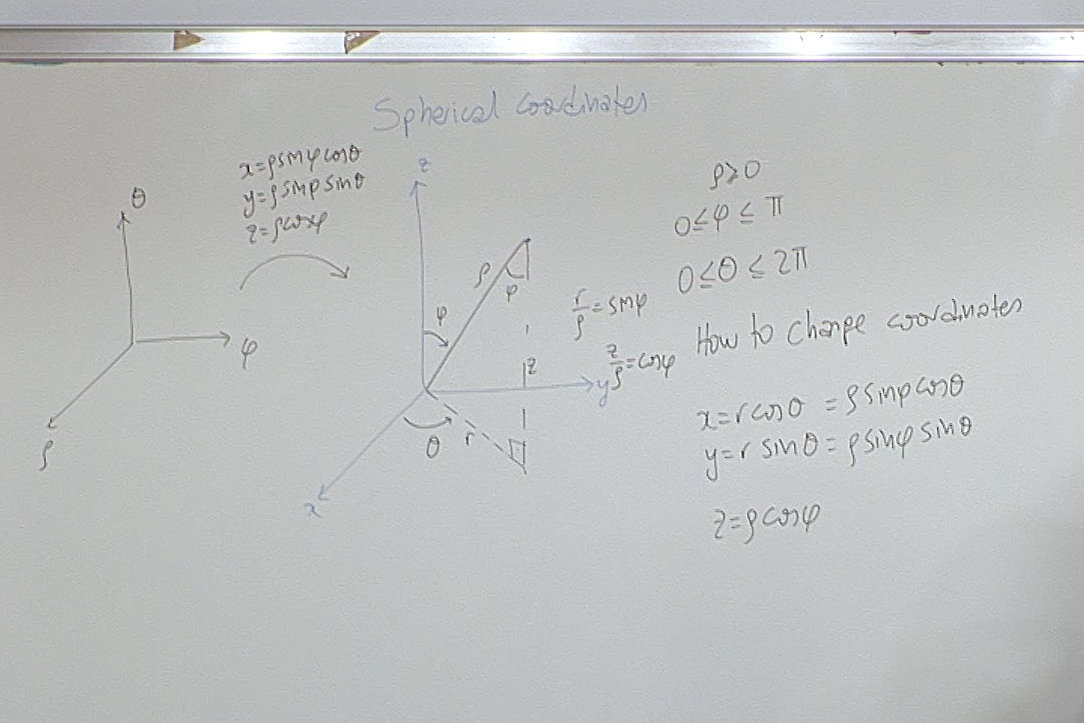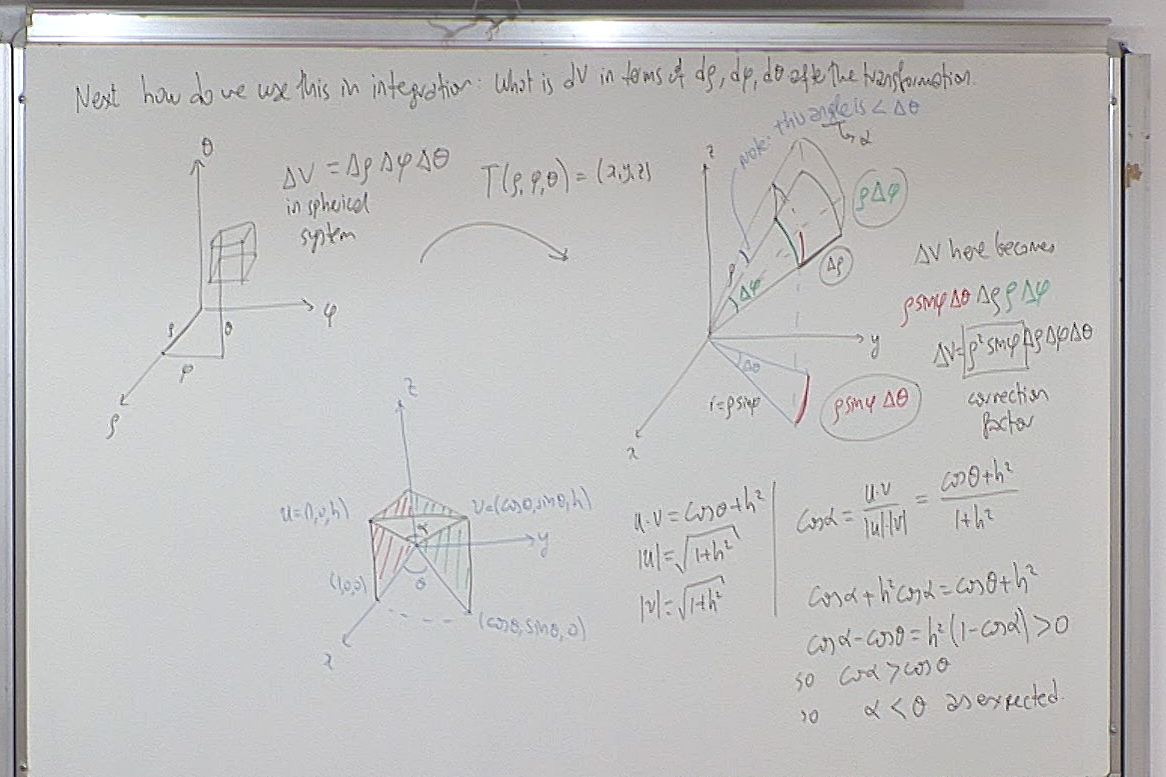On the left we have an incremental box of volume $\Delta\rho\, \Delta \phi\, \Delta \theta$. When this is transformed to cartesian coordinates, its volume becomes $\rho^2\sin\phi\, \Delta\rho\, \Delta \phi\, \Delta \theta$. It is this computation that is explained above.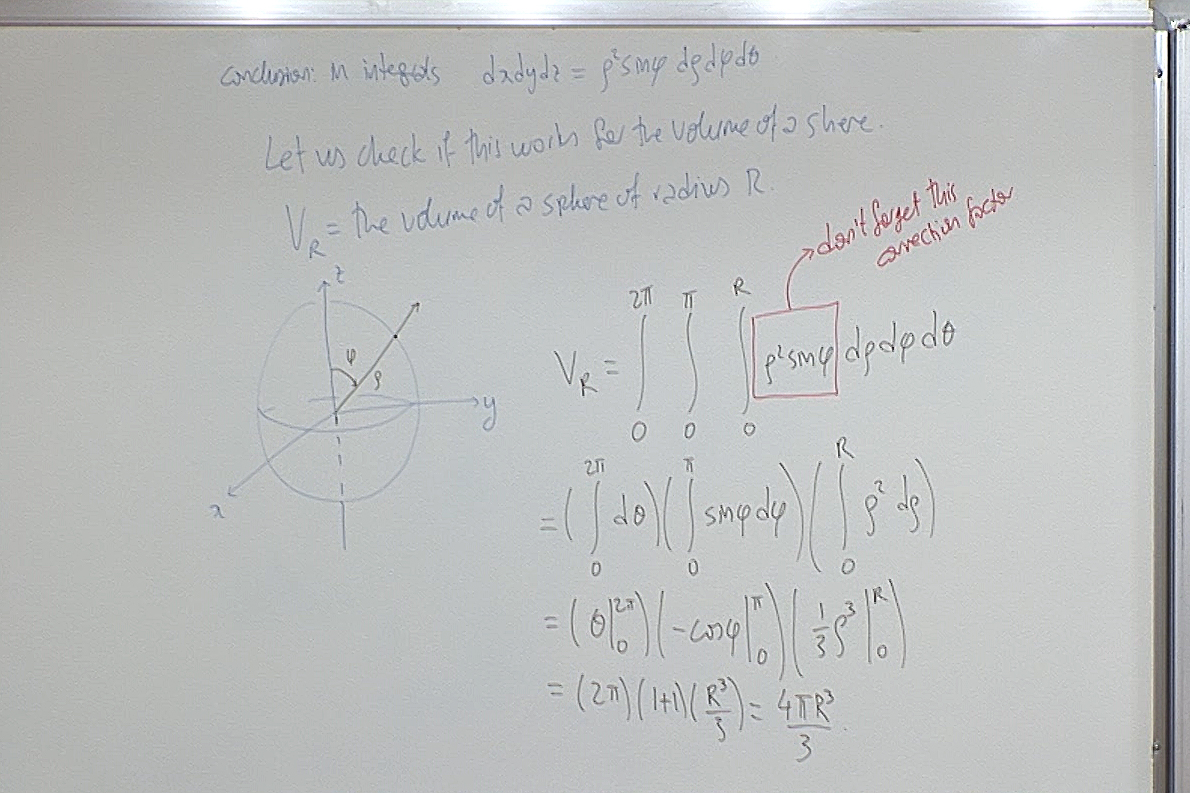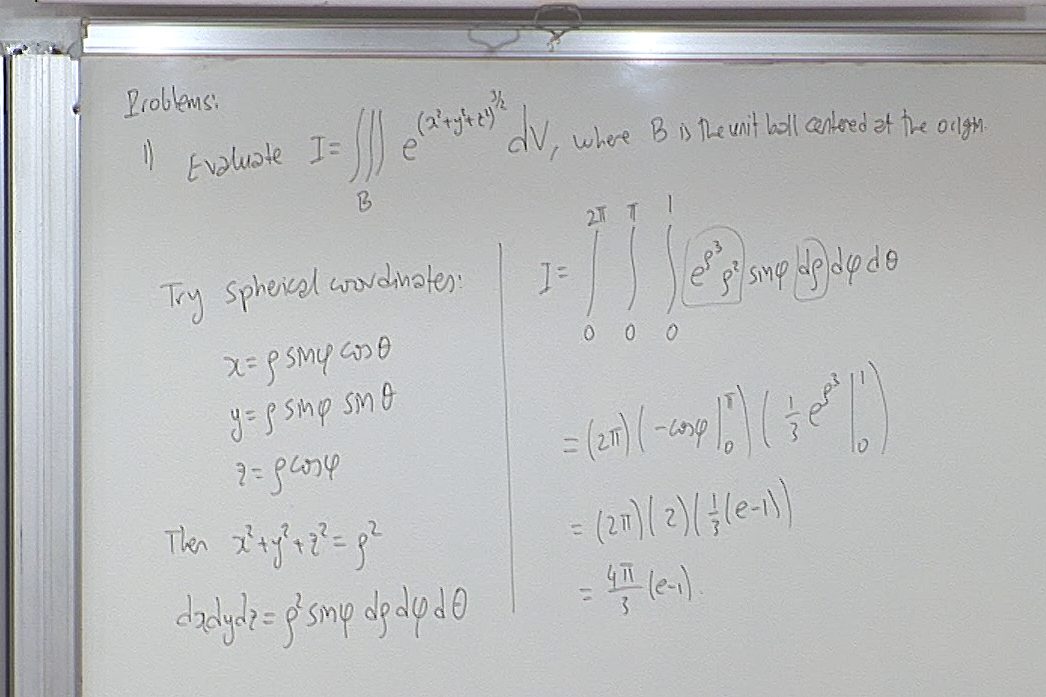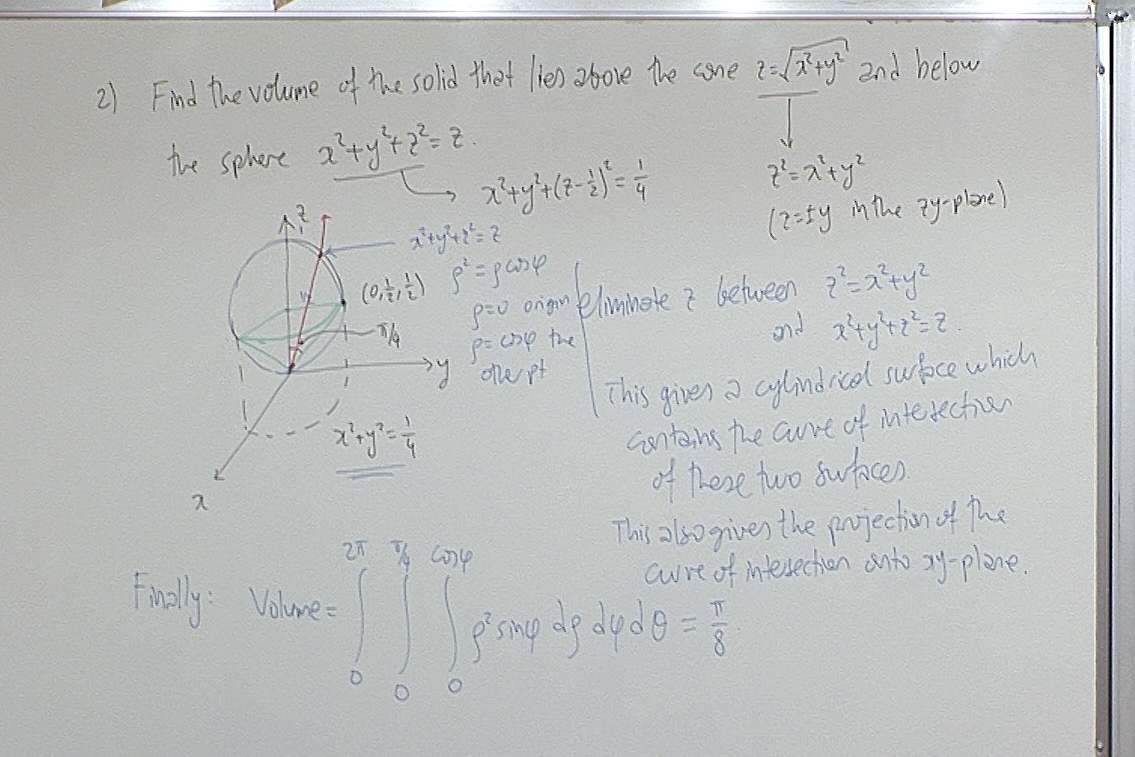For questions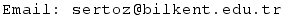Previous Screenshot       Next Screenshot